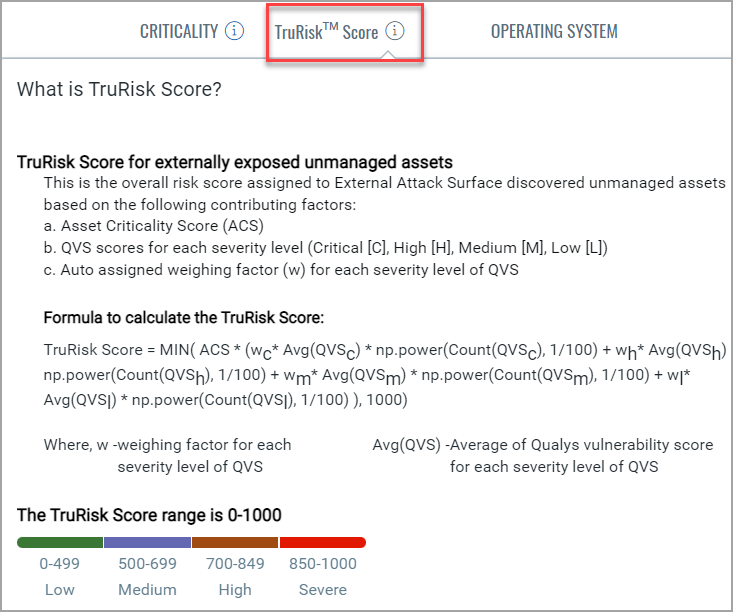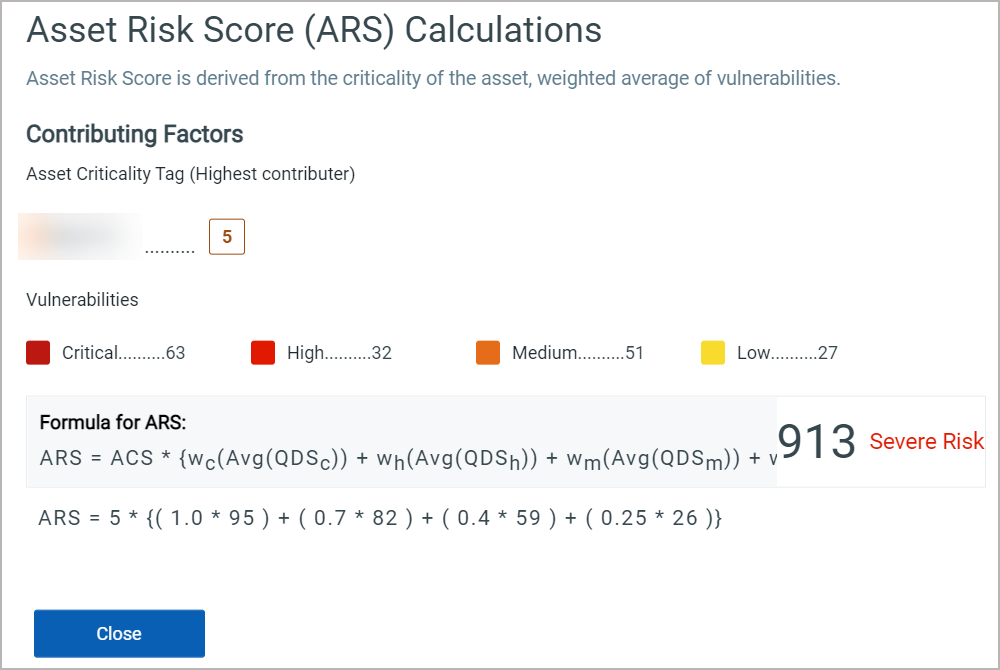# Calculating TruRisk Score

The calculation of TruRisk Score involves various parameters like, Asset Criticality, Qualys Detection Score (QDS), and Qualys Vulnerability Score (QVS). This section informs you about TruRisk Score calculation using these various parameters.

Note: Asset Risk Score is renamed to TruRisk Score.

## Understanding Asset Criticality Score

It is calculated based on multiple tags assigned to the asset with Asset Criticality Scores (ACS) defined. The highest score is considered for the ACS if multiple tags are assigned to the asset.

For example, if you have assigned 6 tags to your asset, the tag with the highest value between 1-5 will be considered as the contributing factor while calculating the TruRisk Score.

## Understanding the Qualys Detection Score

The Qualys Detection Score (QDS) is assigned to vulnerabilities detected by Qualys. QDS has a range from 1 to 100 and with four severity levels:

- Critical: 90-100

- High: 70-89

- Medium: 40-69

- Low: 1-39

QDS is derived from the following factors:

a) Vulnerability technical details (CVSS score): The highest Qualys Vulnerability Score (QVS) for CVEs is associated with the QID.

b) Vulnerability temporal details: Monitors external threat intelligence details for a vulnerability and collect data like Exploit Code Maturity (ECM), malware, active threat actors, and if a threat is trending.

c) Vulnerability remediation details (CIDs): Applies mitigation controls to mitigate the risk from the vulnerability. Vulnerabilities that have applied mitigation controls via Qualys compliance modules will have reduced risk scores.

Note: If multiple CVEs contribute to a QID, the CVE with the highest score is considered for the QDS calculation.

### Understanding the Qualys Vulnerability Score for CVEs

Qualys Vulnerability Score (QVS) is a Qualys-assigned score for a vulnerability based on multiple factors associated with the CVE such as CVSS and external threat indicators like active exploitation, exploit code maturity, CISA known exploitable and many more.

Qualys offers various mitigation controls (CIDs) that are applied to the QVS. Applying all the CIDs to a QID will reduce the QVS. If no CID is applied the QVS will be equal to the QDS.

The following formula is used to calculate the QDS:

QDS = QVS - CID

## Understanding TruRisk Score

TruRisk Score is the overall risk score assigned to the asset based on the following contributing factors:

a) Asset Criticality Score (ACS)

b) Qualys Detection Score (QDS) scores for each QID level

c) Auto-assigned weighting factor (w) for each criticality level of QIDs

The new TruRisk formula includes the number of vulnerabilities; the asset with greater vulnerabilities gets a higher score. The new TruRisk formula has the following features:

- The weighing factor (w) is based on the severity of the vulnerability.

- The maximum risk score restricts to 1000.

- The new formula lists the External Tags.

- In case of an external asset, the entire TruRisk Score value is multiplied with 1.2

The following new TruRisk Score formula is used if an asset is scanned:

 TruRisk Score = MIN( ACS * (wc*Avg(QDSc)*np.power(Count(QDSc), 1/100) `+ ` ```wh*Avg(QDSh)*np.power(Count(QDSh), 1/100)+ ``` ```wm*Avg(QDSm)*np.power(Count(QDSm), ````1/100)+ ` ```wl*Avg(QDSl)*np.power(Count(QDSl), 1/100)),1000)```` `The following old ARS formula is used if an asset is not scanned:

 ARS = ACS * {wc(Avg(QDc)) + wh(Avg(QDSh)) + wm(Avg(QDSm)) + wl(Avg(QDSl))}

where,

ACS - Asset Criticality Score.

w - weighing factor for each severity level of QIDs [critical(c), high(h), medium(m), low(l)]

Avg(QDS) - Average of Qualys risk score for each severity level of QIDs on that asset

np.power - value of np.power is constant to 0.01

Click on the risk score for a particular asset to view the detailed calculation.## Related Topics

TruRisk Score Range in Multi-Grouped Table widget